## Abstract

We examine general decision problems with loss functions that are bounded below. We allow the loss function to assume the value $$\infty$$. No other assumptions are made about the action space, the types of data available, the types of non-randomized decision rules allowed, or the parameter space. By allowing prior distributions and the randomizations in randomized rules to be finitely-additive, we prove very general complete class and minimax theorems. Specifically, under the sole assumption that the loss function is bounded below, we show that every decision problem has a minimal complete class and all admissible rules are Bayes rules. We also show that every decision problem has a minimax rule and a least-favorable distribution and that every minimax rule is Bayes with respect to the least-favorable distribution. Some special care is required to deal properly with infinite-valued risk functions and integrals taking infinite values.

This is a preview of subscription content, access via your institution.

## References

• Battigalli P, Cerreia-Vioglio S, Maccheroni F, Marinacci M (2016) A note on comparative ambiguity aversion and justifiability. Econometrica 84:1903–1916

• Berger JO, Srinivasan C (1978) Generalized bayes estimators in multivariate problems. Ann Stat 6:783–801

• Brown LD (1971) Admissible estimators, recurrent diffusions, and insoluble boundary value problems. Ann Math Stat 42:855–903

• Comfort WW, Negrepontis S (1974) The theory of ultrafilters. Springer, New York

• de Finetti B (1974) Theory of probability. Wiley, New York

• Dunford N, Schwartz JT (1957) Linear operators. Part I: general theory. Wiley, Hoboken

• Heath D, Sudderth W (1972) On a theorem of de finetti, oddsmaking, and game theory. Ann Math Stat 43:2072–2077

• Heath D, Sudderth W (1978) On finitely additive priors, coherence, and extended admissibility. Ann Stat 6:333–345

• Kelley JL (1955) General topology. Springer, New York

• Lehmann EL, Casella G (1998) Theory of point estimation. Springer, New York

• Pearce D (1984) Rationalizable strategic behavior and the problem of perfection. Econometrica 52:1029–1050

• Royden HL (1968) Real analysis. Macmillan, London

• Schervish MJ, Seidenfeld T, Kadane JB (2009) Proper scoring rules, dominated forecasts, and coherence. Decis Anal 6:202–221

• Schervish MJ, Seidenfeld T, Kadane JB (2014) Infinite previsions and finitely additive expectations, 2014. Online supplement to “Dominating countably many forecasts”. Ann Stat 42:728–756. https://doi.org/10.1214/14-AOS1203

• Seidenfeld T, Schervish MJ, Kadane JB (2001) Improper regular conditional distributions. Ann Probab 29:1612–1624. Correction 34:423–426 (2006)

• Wald A, Wolfowitz J (1951) Two methods of randomization in statistics and the theory of games. Ann Math 52:581–586

• Willard S (1970) General topology. Addison-Wesley, Boston

## Acknowledgements

The authors wish to offer thanks to two excellent reviewers and the editors for their invaluable help and limitless patience in the processing of this article.

## Author information

Authors

### Corresponding author

Correspondence to Mark J. Schervish.

### Publisher's Note

Springer Nature remains neutral with regard to jurisdictional claims in published maps and institutional affiliations.

## Electronic supplementary material

Below is the link to the electronic supplementary material.

## Appendices

### Unbounded and infinite-valued functions

In de Finetti (1974), de Finetti laid out the theory of coherent previsions for bounded random variables, which he related to finitely-additive probability. De Finetti’s definition of coherent prevision is the following.

### Definition 7

Let $$\mathcal{F}$$ be a set of bounded functions defined on a space $$\mathcal{Z}$$. For each $$f\in \mathcal{F}$$, let P(f) be a real number. De Finetti called $$\{P(f):f\in \mathcal{F}\}$$coherent previsions for $$\mathcal{F}$$ if, for every finite integer n, every n-tuple $$(f_1,\ldots ,f_n)\in \mathcal{F}^n$$, and every n-tuple $$(\alpha _1,\ldots ,\alpha _n)$$ of real numbers,

\begin{aligned} \sup _z\sum _{j=1}^n\alpha _j[f_j(z)-P(f_j)]\ge 0. \end{aligned}
(13)

If $$\mathcal{F}$$ consists solely of indicator functions of events, coherent previsions for $$\mathcal{F}$$ can be shown to form a finitely-additive probability. (See de Finetti 1974, Chapter 3 for a long-winded, but elementary treatment.) Also, a finitely-additive probability can be defined on an arbitrary collection of subsets of a general set $$\mathcal{Z}$$, including the power set. A number of theorems in Bhaskara Rao and Bhaskara Rao (1983, Chapter 3) show how to extend a partially defined finitely-additive probability to arbitrary larger domains. Theorems 3.2.9 and 3.2.10 on pages 69–70 of Bhaskara Rao and Bhaskara Rao (1983) are very general. For this reason, measurability conditions are often not included in theorems about finitely-additive probabilities. In this paper, we assume that each finitely-additive probability is defined on $$2^\mathcal{Z}$$. If the reader starts with a finitely-additive probability P defined on a smaller domain, our results will apply to every extension of P to $$2^\mathcal{Z}$$.

Extending a finitely-additive probability P defined on $$2^\mathcal{Z}$$ to a coherent prevision on the set of all bounded functions in $$\mathcal{M}_\mathcal{Z}$$ is straightforward. For each simple function $$f=\sum _{j=1}^n\alpha _jI_{A_j}$$ on $$\mathcal{Z}$$ (with all $$\alpha _j$$ finite), there is a unique value for the coherent previson $$P(f)=\sum _{j=1}^n\alpha _jP(A_j)$$. (See Bhaskara Rao and Bhaskara Rao 1983, Proposition 4.4.2 on page 97.) Because every bounded function is uniformly approximable both above and below by simple functions, for each bounded function f on $$\mathcal{Z}$$, there is a unique value for the finitely-additive expectation

\begin{aligned} P(f)=\sup _{_{\text{ simple } g\le f}}P(g)=\inf _{_{\text{ simple } g\ge f}}P(g). \end{aligned}

In this paper, we need an extension of the finitely-additive theory from bounded functions both to unbounded functions, as was done by Schervish et al. (2014), and to functions that assume the value $$\infty$$ as well. The extension to unbounded functions in Schervish et al. (2014) generalizes the concept of coherence to allow $$P(f)=\infty$$ without requiring the symbol $$\infty$$ to appear in (13). They then prove in Schervish et al. (2014, Definition 7, Lemmas 4 and 6) that previsions are coherent if and only if they are the values of a normalized monotone linear functional. The assumption that the loss function is bounded below allows us to avoid dealing with functions that both assume the value $$\infty$$ and are unbounded below. This has the added benefit of allowing us to avoid arithmetic that leads to $$\infty -\infty$$.

Because the set $$\mathcal{M}_\mathcal{Z}$$ of functions that are bounded below is not a linear space, we need to generalize the concept of normalized monotone linear functional.

### Definition 8

Let $$\mathcal{Z}$$ be a space, and let $$\mathcal{F}$$ be a subset of $$\mathcal{M}_\mathcal{Z}$$ that contains all constant functions and has the following restricted linearity property: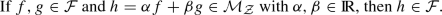(14)

We call such a set $$\mathcal{F}$$ a restricted-linear space. If $$\mathcal{F}$$ is a restricted-linear space, a functionthat satisfies

\begin{aligned} L(\alpha f+\beta g)=\alpha L(f)+\beta L(g), \end{aligned}
(15)

whenever $$f,g,\alpha f+\beta g\in \mathcal{F}$$ and the arithmetic on the right-hand side of (15) is well defined (i.e., does not involve $$\infty -\infty$$,) is called a restricted-linear functional. A restricted-linear functional is called monotone if $$f\le g$$ implies $$L(f)\le L(g)$$. A monotone restricted-linear functional is called a finitely-additive Daniell integral. If $$L(1)=1$$, we say that L is normalized. A normalized finitely-additive Daniell integral is called a finitely-additive expectation.

Note that $$\mathcal{M}_\mathcal{Z}$$ is a restricted-linear space, as is the set of bounded functions. The unique coherent prevision on the bounded functions constructed above from a finitely-additive probability on $$2^\mathcal{Z}$$ is an example of a finitely-additive expectation.

The various conditions in Definition 8 prevent $$\infty -\infty$$ from appearing on either side of (15). Note that one cannot have $$\alpha$$ and $$\beta$$ both negative in (14) unless both f and g are bounded. If at least one of the functions fg is unbounded in (14), then at least one of the unbounded functions must have a positive coefficient in order for the linear combination to be well-defined and bounded below. Examples of each of the following situations arise, and they are the reason that we do not enforce (15) when the arithmetic on the right-hand side involves $$\infty -\infty$$:

• The difference between two functions that are unbounded above can be bounded below.

• The difference of two functions, each with infinite finitely-additive expectation, can have finite expectation.

Although there is no unique extension of a finitely-additive expectation from the set of bounded functions to $$\mathcal{M}_\mathcal{Z}$$, there are two special extensions that exist and prove useful. Lemmas 9 and 10 apply to unbounded and infinite-valued functions. Equation 1.2 of Heath and Sudderth (1978) states a result like Lemma 9 without proof and without being explicit about the fact that the extension might take the value $$\infty$$. The restriction to functions that are bounded below is important (even for the result of Heath and Sudderth 1978) in order to avoid $$\infty -\infty$$.

### Lemma 9

Let $$\mathcal{J}$$ be the set of all bounded real-valued functions defined on a set $$\mathcal{Z}$$, and let P be a finitely-additive expectation on $$\mathcal{J}$$. For each $$f\in \mathcal{M}_\mathcal{Z}$$, define

\begin{aligned} Q(f)=\sup _{_{g\in \mathcal{J}\text{, } g\le f}}P(g). \end{aligned}

Then $$Q=P$$ on $$\mathcal{J}$$, and Q is a finitely-additive expectation on $$\mathcal{M}_\mathcal{Z}$$.

### Proof

Because P is a finitely-additive expectation, $$g\le f$$ implies $$P(g)\le P(f)$$ for $$f,g\in \mathcal{J}$$. Hence $$Q(f)=P(f)$$ for $$f\in \mathcal{J}$$.

Next, we show that Q is restricted-linear. Let $$f_1,f_2\in \mathcal{M}_\mathcal{Z}$$,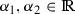, and $$\alpha _1f_1+\alpha _2f_2\in \mathcal{M}_\mathcal{Z}$$. We need to show that $$Q(\alpha _1f_1+\alpha _2f_2)=\alpha _1Q(f_1)+\alpha _2Q(f_2)$$ whenever the arithmetic on the right-hand side is well-defined. Since $$Q(\alpha _jf_j)=\alpha _jQ(f_j)$$ if $$\alpha _j>0$$, there is no loss of generality in assuming that $$\alpha _1,\alpha _2$$ are each either 1 or $$-1$$. First, we show that $$Q(f_1+f_2)=Q(f_1)+Q(f_2)$$ for $$f_1,f_2\in \mathcal{M}_\mathcal{Z}$$. Notice that $$Q(f)=\lim _{m\rightarrow \infty }P(f\wedge m)$$ because every bounded $$g\le f$$ is bounded above by $$f\wedge m$$ where $$m=\sup _z g(z)$$. Then notice that, for all m,

\begin{aligned} (f_1\wedge m/2)+(f_2\wedge m/2)\le (f_1+f_2)\wedge m\le (f_1\wedge m)+(f_2\wedge m). \end{aligned}

The limits of the left-hand and right-hand expressions are both $$Q(f_1)+Q(f_2)$$ while the limit of the middle expression is $$Q(f_1+f_2)$$. To complete the proof that Q is restricted-linear, we need to show that $$Q(f_1-f_2)=Q(f_1)-Q(f_2)$$ if $$f_1-f_2\in \mathcal{M}_\mathcal{Z}$$ and $$Q(f_2)$$ is finite. What we just proved implies that

\begin{aligned} Q(f_1)=Q(f_1-f_2+f_2)=Q(f_1-f_2)+Q(f_2). \end{aligned}
(16)

Since $$Q(f_2)$$ is finite, we can subtract it from both sides of (16) to complete this part of the proof.

Next, we show that Q is monotone. Let $$f,g\in \mathcal{M}_\mathcal{Z}$$ with $$f\le g$$. We need to show that $$Q(f)\le Q(g)$$. Define

\begin{aligned} g'(z)=\left\{ \begin{array}{ll} g(z)-f(z)&{}\quad \text{ if } f(z)<\infty \text{, }\\ 0&{}\quad \text{ otherwise. }\end{array}\right. \end{aligned}

Then $$g=f+g'$$, $$g'\ge 0$$, and $$g'\in \mathcal{M}_\mathcal{Z}$$. Hence, $$0\le Q(g')$$ from the definition of Q. From the previous part of the proof,

\begin{aligned} Q(g)=Q(f+g')=Q(f)+Q(g')\ge Q(f). \end{aligned}

$$\square$$

At the other extreme from Lemma 9, we have the following alternative extension of a finitely-additive expectation from bounded functions to $$\mathcal{M}_\mathcal{Z}$$.

### Lemma 10

Assume the conditions of Lemma 9. Define L on $$\mathcal{M}_\mathcal{Z}$$ as follows: $$L(f)=P(f)$$ if $$f\in \mathcal{J}$$, and $$L(f)=\infty$$ if $$f\in \mathcal{M}_\mathcal{Z}{\setminus }\mathcal{J}$$. Then L is a finitely-additive expectation on $$\mathcal{M}_\mathcal{Z}$$ that extends P.

### Proof

Clearly, L extends P. Also, L is monotone since every instance of $$f\le g$$ either has both $$f,g\in \mathcal{J}$$ or $$L(g)=\infty$$. For (15), if either $$\alpha$$ or $$\beta$$ is 0, the equation holds. When both $$\alpha$$ and $$\beta$$ are nonzero, consider four cases:

1. (i)

$$\alpha f,\beta g\in \mathcal{J}$$. In this case both sides of (15) are the same because P is linear on $$\mathcal{J}$$.

2. (ii)

$$\alpha f\in \mathcal{J}$$ and $$\beta g\not \in \mathcal{J}$$. In this case, $$\beta >0$$ so that both sides of (15) are $$\infty$$.

3. (iii)

$$\alpha f\not \in \mathcal{J}$$ and $$\beta g\in \mathcal{J}$$. In this case, $$\alpha >0$$ so that both sides of (15) are $$\infty$$.

4. (iv)

$$\alpha f,\beta g\not \in \mathcal{J}$$. In this case, at least one of $$\alpha$$ or $$\beta$$ must be positive. If $$\alpha$$ and $$\beta$$ are both positive, both sides of (15) are $$\infty$$. If one of them is negative, the right-hand side of (15) is $$\infty -\infty$$, and (15) has no force.

$$\square$$

### Definition 9

We refer to Q in Lemma 9 as the minimum extension of P. If a prevision Q is the minimum extension of its restriction to the bounded random variables, then we say that Q is a minimum extension. We refer to the L in Lemma 10 as the maximum extension of P. We also use the notation $$\int _\mathcal{Z}f(z)P(dz)$$ to stand for Q(f) because Q(f) is a finitely-additive Daniell integral and is determined uniquely from P and f.

The terms “minimum extension” and “maximum extension” are used for Q and L respectively because they assign the minimum and maximum of all possible finitely-additive expectations that extend P to $$\mathcal{M}_\mathcal{Z}$$.

It is also straightforward to see that the measure-theoretic definition of expectation with respect to a countably-additive probability P is the restriction of a minimum extension to the functions in $$\mathcal{M}_\mathcal{Z}$$ that are measurable with respect to the $$\sigma$$-field on which P is defined. Nothing in the countably-additive theory corresponds to the maximum extension. There are extensions that are neither minimum nor maximum, but these cannot generally be constructed in one step, like we did for the minimum and maximum extensions.

The following is a generalization of a well-know measure-theoretic result.

### Lemma 11

Let $$\mathcal{Z}$$ and $$\mathcal{Y}$$ be sets. Let P be a finitely-additive expectation on $$\mathcal{M}_\mathcal{Y}$$. Let $$h:\mathcal{Y}\rightarrow \mathcal{Z}$$ be a function. Then $$P'$$ defined by $$P'(f)=P[f(h)]$$ is a finitely-additive expectation defined on $$\mathcal{M}_\mathcal{Z}$$ which we call the finitely-additive expectation induced by h.

### Proof

Clearly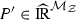, and $$P'(1)=P(1)=1$$. If $$f\le g$$, then $$f(h)\le g(h)$$ and $$P'(f)\le P'(g)$$. We need to show that $$P'$$ is extended-linear. Assume that $$f,g,\alpha f+\beta g\in \mathcal{M}_\mathcal{Z}$$ and $$\alpha P'(f)$$ and $$\beta P'(g)$$ are not both infinite of opposite signs. Then $$\alpha P[f(h)]$$ and $$\beta P[g(h)]$$ are not both infinite of opposite signs and $$P[\alpha f(h)+\beta g(h)]=\alpha P[f(h)]+\beta P[g(h)]$$, which implies $$P'(\alpha f+\beta g)=\alpha P'(f)+\beta P'(g)$$. $$\square$$

### Definition 10

Let S be a set and let $$\mathcal{U}$$ be a non-empty collection of subsets of S. We call $$\mathcal{U}$$ an ultrafilter on S if (i) $$A\in \mathcal{U}$$ and $$A\subseteq B$$ implies $$B\in \mathcal{U}$$, (ii) $$A,B\in \mathcal{U}$$ implies $$A\cap B\in \mathcal{U}$$, and (iii) for every $$A\subseteq S$$, $$A\in \mathcal{U}$$ if and only if $$A^C\not \in \mathcal{U}$$. An ultrafilter on S is principal if there exists $$s\in S$$ such that $$\mathcal{U}$$ consists of all subset of S that contain s. Such an s is called the atom of $$\mathcal{U}$$. Other ultrafilters are called non-principal.

Proposition 9 gives general conditions under which ultrafilters exist. It requires a definition first.

### Definition 11

A collection $$\mathcal{F}$$ of subsets of a set $$\mathcal{Z}$$ has the finite-intersection property if every finite subcollection has non-empty intersection.

### Proposition 9

(Comfort and Negrepontis 1974: Special case of Theorem 2.18, p. 39) Let $$\mathcal{Z}$$ be a set, and let $$\mathcal{F}$$ be a collection of subsets. If $$\mathcal{F}$$ has the finite intersection property, then there is an ultrafilter on $$\mathcal{Z}$$ that contains $$\mathcal{F}$$.

We have two main uses for ultrafilters in this paper. One is to use them as examples of merely finitely-additive probabilities via Lemma 12 below. The other is to exploit the connection between limits of nets and integrals with respect to ultrafilter probabilities defined on directed sets via Lemma 14 below.

There is a correspondence between ultrafilters and 0–1-valued probabilities.

### Lemma 12

Let $$\mathcal{Z}$$ be a set, and let $$\mathcal{F}$$ be a field of subsets of $$\mathcal{Z}$$. A finitely-additive probability P defined on $$\mathcal{F}$$ takes only the values 0 and 1 if and only if (i) P can be extended to $$P'$$ defined on $$2^\mathcal{Z}$$ and (ii) there is an ultrafilter $$\mathcal{U}$$ of subsets of $$\mathcal{Z}$$ such that $$P'(E)=1$$ if and only if $$E\in \mathcal{U}$$.

### Proof

For the “if” direction, the restriction of $$P'$$ to $$\mathcal{F}$$ takes only the values 0 and 1. For the “only if” direction, $$\mathcal{V}=\{E\in \mathcal{F}: P(E)=1\}$$ has the finite-intersection property, hence there is an ultrafilter $$\mathcal{U}$$ of subsets of $$\mathcal{Z}$$ such $$\mathcal{V}\subseteq \mathcal{U}$$. Define $$P'(E)=1$$ if $$E\in \mathcal{U}$$ and $$P'(E)=0$$ if $$E\not \in \mathcal{U}$$. It is clear that $$P'$$ is finitely-additive on $$2^\mathcal{Z}$$. $$\square$$

### Definition 12

Let $$\mathcal{U}$$ be an ultrafilter of subsets of some set $$\mathcal{Z}$$. We call the probability P defined by $$P(E)=1$$ if $$E\in \mathcal{U}$$ and $$P(E)=0$$ if $$E\not \in \mathcal{U}$$ the probability corresponding to$$\mathcal{U}$$.

### Lemma 13

Let $$\mathcal{D}$$ be a set. Let $$\mathcal{U}$$ be an ultrafilter of subsets of $$\mathcal{D}$$. Let P be the minimum extension of the probability on $$\mathcal{D}$$ corresponding to $$\mathcal{U}$$. Then, for each $$f\in \mathcal{M}_\mathcal{D}$$,

\begin{aligned} \int _\mathcal{D}f(\eta )P(d\eta )=\sup _{B\in \mathcal{U}}\inf _{\eta \in B}f(\eta )= \inf _{B\in \mathcal{U}}\sup _{\eta \in B}f(\eta ). \end{aligned}

### Proof

Let $$k_0=\int _\mathcal{D}f(\eta )P(d\eta )$$. Since (i) $$P(B)=1$$ for all $$B\in \mathcal{U}$$, (ii) P is a monotone restricted-linear functional, and (iii) P is a minimum extension, $$k_0\le \sup _{\eta \in B}f(\eta )$$, for all $$B\in \mathcal{U}$$. Similarly, $$k_0\ge \inf _{\eta \in B}f(\eta )$$, for all $$B\in \mathcal{U}$$. It follows that

\begin{aligned} \sup _{B\in \mathcal{U}}\inf _{\eta \in B}f(\eta )\le k_0\le \inf _{B\in \mathcal{U}}\sup _{\eta \in B}f(\eta ). \end{aligned}
(17)

If the two endpoints, call them $$a\le b$$, of (17) are not equal, then for each $$c\in (a,b)$$ precisely one of $$\{\eta :f(\eta )\le c\}$$ or $$\{\eta :f(\eta )>c\}$$ is in $$\mathcal{U}$$. If it is the first of these, it contradicts $$b=\inf _{B\in \mathcal{U}}\sup _{\eta \in B}f(\eta )$$. If it is the second one, it contradicts $$a=\sup _{B\in \mathcal{U}}\inf _{\eta \in B}f(\eta )$$. Hence $$a=b$$, and both inequalities in (17) are equality. $$\square$$

The final result requires a definition first.

### Definition 13

Let $$x=\{x_\eta \}_{\eta \in \mathcal{D}}$$ be a net in a topological space $$\mathcal{T}$$. We call a net $$y=\{y_\gamma \}_{\gamma \in \mathcal{D}'}$$ a subnet of x if there exists a function $$h:\mathcal{D}'\rightarrow \mathcal{D}$$ with the following properties: (i) $$y_\gamma =x_{h(\gamma )}$$ for all $$\gamma \in \mathcal{D}'$$, (ii) $$\gamma _1\le _{\mathcal{D}'}\gamma _2$$ implies $$h(\gamma _1)\le _{\mathcal{D}}h(\gamma _2)$$ and (iii) for every $$\eta \in \mathcal{D}$$ there exists $$\gamma \in \mathcal{D}'$$ such that $$h(\gamma )\ge _\mathcal{D}\eta$$. A cluster point of x is a point $$p\in \mathcal{T}$$ such that, for every neighborhood N of p and every $$\eta \in \mathcal{D}$$, there exists $$\eta '\in \mathcal{D}$$ such that $$\eta '\ge _\mathcal{D}\eta$$ and $$x_{\eta '}\in N$$.

See Section 1 of the Online Resource for more detail about nets and subnets.

### Lemma 14

Let $$\mathcal{Z}$$ be a set. Let $$\mathcal{D}$$ be a directed set and let $$f=\{f_\eta \}_{\eta \in \mathcal{D}}$$ be a net in $$\mathcal{M}_\mathcal{Z}$$. An element g of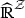is a cluster point of f if and only if there is an ultrafilter $$\mathcal{U}$$ that contains all tails of $$\mathcal{D}$$ whose corresponding probability on $$\mathcal{D}$$ has minimum extension P such that $$g(z)=\int _\mathcal{D}f_\eta (z)P(d\eta )$$ for all $$z\in \mathcal{Z}$$.

### Proof

For the “only if” direction, assume first that f converges to g. Let $$\mathcal{U}$$ be any ultrafilter that contains all tails of $$\mathcal{D}$$, and let P be the minimum extension of the corresponding probability on $$\mathcal{D}$$. Let $$h(z)=\int _\mathcal{D}f_\eta (z)P(d\eta )$$ for each $$z\in \mathcal{Z}$$. We need to show that, for each $$z\in \mathcal{Z}$$ and each neighborhood N of g(z), $$h(z)\in N$$. Let $$z\in \mathcal{Z}$$. Lemma 13, applied to ultrafilters of subsets of $$\mathcal{D}$$, says that

\begin{aligned} \sup _{B\in \mathcal{U}}\inf _{\eta \in B}f_\eta (z)= h(z)= \inf _{B\in \mathcal{U}}\sup _{\eta \in B}f_\eta (z). \end{aligned}
(18)

If g(z) is finite, let $$\epsilon >0$$, and let N be the interval $$(g(z)-\epsilon ,g(z)+\epsilon )$$. Because f converges to g, there exists $$\eta \in \mathcal{D}$$ such that $$f_\beta (z)\in N$$ for all $$\beta \ge _\mathcal{D}\eta$$. Let $$B=A_\eta =\{\beta \in \mathcal{D}:\eta \le _\mathcal{D}\beta \}$$, which is in $$\mathcal{U}$$. It follows that the left and right sides of (18) are respectively at least $$g(z)-\epsilon$$ and at most $$g(z)+\epsilon$$. Hence $$h(z)\in N$$. If $$g(z)=\infty$$, let $$N=(c,\infty ]$$. Then there exists $$\eta \in \mathcal{D}$$ such that $$f_\beta (z)>c$$ for all $$\beta \ge _\mathcal{D}\eta$$. Let $$B=A_\eta$$, which is in $$\mathcal{U}$$. It follows that the left side of (18) is at least c, so that $$h(z)\in N$$. Next, assume that g is merely a cluster point of f. By Proposition 6, there exists a subnet $$f'=\{r_\tau \}_{\tau \in \mathcal{D}'}$$ that converges to g. The previous argument shows that for every ultrafilter $$\mathcal{U}'$$ on $$\mathcal{D}'$$ that contains all tails of $$\mathcal{D}'$$, $$g(z)=\int _{\mathcal{D}'}r_\tau (z)P'(d\tau )$$, where $$P'$$ is the probability corresponding to $$\mathcal{U}'$$. Let $$h:\mathcal{D}'\rightarrow \mathcal{D}$$ be the function in Definition 13 that embeds $$\mathcal{D}'$$ into $$\mathcal{D}$$. Then $$r_\tau =f_{h(\tau )}$$, so that $$g(z)=\int _{\mathcal{D}'}f_{h(\tau )}(z)P'(d\tau )$$. Let P be the probability on $$\mathcal{D}$$ induced by h from $$P'$$ (Lemma 11.) Then

\begin{aligned} \int _{\mathcal{D}}f_\eta (z)P(d\eta )=\int _{\mathcal{D}'}f_{h(\tau )}(z)P'(d\tau ). \end{aligned}

For the “if” direction, let $$\mathcal{U}$$ be an ultrafilter that contains all tails of $$\mathcal{D}$$ such that $$g(z)=\int _\mathcal{D}f_\eta (z)P(d\eta )$$ for all $$z\in \mathcal{Z}$$, where P is the minimum extension of the probability on $$\mathcal{D}$$ corresponding to $$\mathcal{U}$$. We need to show that g is a cluster point of f. Specifically, we need to show that, for every neighborhood N of g and $$\eta \in \mathcal{D}$$, there exists $$\beta \ge _\mathcal{D}\eta$$ such that $$f_\beta \in N$$. It suffices to prove this claim for all neighborhoods of the form $$N=\{h:h(z_j)\in N_j, \text{ for } j=1,\ldots ,n\}$$, for arbitrary positive integer n, distinct $$z_1,\ldots ,z_n\in \mathcal{Z}$$ and neighborhoods $$N_1,\ldots ,N_n$$ of the form $$N_j=(g(z_j)-\epsilon ,g(z_j)+\epsilon )$$ for $$\epsilon >0$$ if $$g(z_j)$$ is finite and $$N_j=(c,\infty ]$$ if $$g(z_j)=\infty$$. So, let N be of the form just described, and let $$\eta \in \mathcal{D}$$. We need to find $$\beta \in \mathcal{D}$$ such that $$\eta \le _\mathcal{D}\beta$$ and $$f_\beta (z_j)\in N_j$$ for $$j=1,\ldots ,n$$. We know that, for all $$z\in \mathcal{Z}$$,

\begin{aligned} \sup _{B\in \mathcal{U}}\inf _{\beta \in B}f_\beta (z)=g(z) =\inf _{B\in \mathcal{U}}\sup _{\beta \in B}f_\beta (z). \end{aligned}

For each j such that $$g(z_j)$$ is finite, let $$B_j\in \mathcal{U}$$ be such that

\begin{aligned} \inf _{\eta \in B_j}f_\beta (z_j)> & {} g(z_j)-\epsilon ,\\ \sup _{\eta \in B_j}f_\beta (z_j)< & {} g(z_j)+\epsilon . \end{aligned}

For each j such that $$g(z_j)=\infty$$, let $$B_j\in \mathcal{U}$$ be such that

\begin{aligned} \inf _{\beta \in B_j}f_\beta (z_j)>c. \end{aligned}

If we replace $$B_j$$ by $$B_j\cap A_\eta$$, all of the last three inequalities above continue to hold. For each j, let $$\beta _j\in B_j$$, and let $$\beta \ge _\mathcal{D}\beta _j$$ for all j. Then $$\eta \le _\mathcal{D}\beta$$ and $$f_\beta (z_j)\in N_j$$ for all j. $$\square$$

### Results whose proofs are in the online resource

This appendix contains results whose proofs are located in the Online Resource.

### Proposition 10

(Lemma 10 in Section 2.3 of the Online Resource) Let $$\mathcal{Z}$$ be a set. Then $$\mathcal{P}_\mathcal{Z}$$ is compact.

### Proposition 11

(Lemma 2 in Section 1.2 of the Online Resource) Let $$\mathcal{Z}$$ be a set. For every $$z\in \mathcal{Z}$$ and every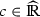,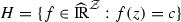is closed. Also, both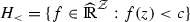and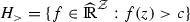are open.

### Proposition 12

(Lemma 18 in Section 3 of the Online Resource) Let G be a closed convex subset of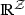consisting of non-negative functions such that $$g\in G$$ and $$f\ge g$$ implies $$f\in G$$. Let $$k\in \partial _L G$$. Then there exists a finitely-additive expectation $$\lambda _k\in \varLambda _\mathcal{Z}$$ such that $$\lambda _k(k)\le \lambda _k(g)$$ for all $$g\in G$$.

### Proposition 13

(Lemma 1 in Section 1.2 of the Online Resource) Let $$\{f_\eta \}_{\eta \in \mathcal{D}}$$ be a convergent net (with limit $$f_0$$) infor some set $$\mathcal{Z}$$.

• If $$\sup _{z\in \mathcal{Z}}f_\eta (z)\le c_\eta$$ for all $$\eta$$ and $$\{c_\eta \}_{\eta \in \mathcal{D}}$$ converges to $$c_0$$, then $$\sup _{z\in \mathcal{Z}}f_0(z)\le c_0$$.

• If $$\inf _{z\in \mathcal{Z}}f_\eta (z)\ge c_\eta$$ for all $$\eta$$ and $$\{c_\eta \}_{\eta \in \mathcal{D}}$$ converges to $$c_0$$, then $$\inf _{z\in \mathcal{Z}}f_0(z)\ge c_0$$.

### Proposition 14

(Corollary 1 in Section 1.2 of the Online Resource) Let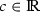. Then $$\{f: \inf _{z\in \mathcal{Z}}f(z)\ge c\}$$ and $$\{f: \sup _{z\in \mathcal{Z}}f(z)\le c\}$$ are closed.

### Proposition 15

(Lemma 17 in Section 3 of the Online Resource) Let G be a closed convex subset ofconsisting of non-negative functions such that $$g\in G$$ and $$f\ge g$$ implies $$f\in G$$. Let k be a real-valued function, and define

\begin{aligned} H_k=\{h\in \mathcal{M}_\mathcal{Z}: h(z)<k(z), \text{ for } \text{ each } \text{ z }\}. \end{aligned}

Suppose that $$H_k\cap G=\emptyset$$. Then there exists $$\lambda _k\in \varLambda _\mathcal{Z}$$ such that $$\lambda _k(k)\le \lambda _k(g)$$ for all $$g\in G$$.

### Proposition 16

(Lemma 22 in Section 5 of the Online Resource) Let $$\mathcal{Z}$$ be a set. A finitely-additive expectation $$\lambda$$ on $$\mathcal{M}_\mathcal{Z}$$ is continuous in the pointwise topology if and only if $$\lambda$$ is simple and is a minimum extension.

### Proposition 17

(Lemma 11 in Section 2.3 of the Online Resource) Let $$\mathcal{Z}$$ be a set. Then $$\mathcal{S}_\mathcal{Z}$$ is dense in $$\varLambda _\mathcal{Z}$$.

### Proposition 18

(Lemma 12 in Section 2.4 of the Online Resource) Let $$\mathcal{X}$$ and $$\mathcal{Y}$$ be sets. Let P be a finitely-additive probability on $$2^\mathcal{Y}$$. Let Q be a countably-additive discrete probability on $$2^\mathcal{X}$$. Then $$P[Q](f)=Q[P](f)$$ for each bounded function f.

## Rights and permissions

Reprints and Permissions